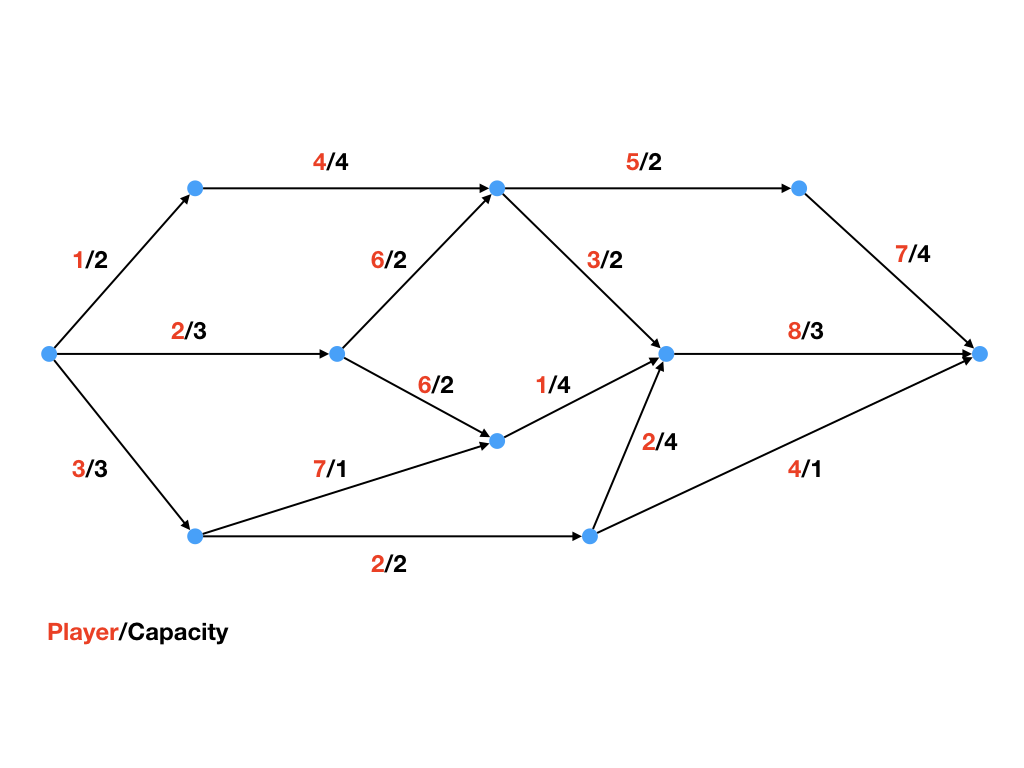Warning

# Assignment #3 - Flow Games

Your goal is to compute the Shapley value of 8 agents controlling the links in this communication network:You need to derive a flow game from the network. For the computation of Shapley value use the sampling algorithm based on the statistical estimation. You can use the template source code we prepared for you.

1. Flow games: 10.01.2020 4:00 CET [12 pts]

# Input

Consider the following:

V is the set of vertices of the graph G.
E is the set of (directed) edges of the graph G.
N is the set of players controlling the edges of G.
E ∋ ei = (u, v), where u, v ∈ V.
c: E → ℜ+ is the capacity function, mapping an edge to a real (upper) capacity value, where ∀e ∈ E : c(e) ≥ 0.
a: E → N is the controlling agent function, mapping an edge to the agent controlling it.
Assume that all lower capacities equal to zero. 

You are provided with an input describing the graph in the following format. Assume m = |E|, and node ids v.id = 0, if v = source and v.id = 1, if v = sink:

|V| |E| |N|
u.id v.id c(e1) a(e1)
...
u.id v.id c(ei) a(ei)
...
u.id v.id c(em) a(em)

# Output

The output of your program must be the estimated Shapley value for each player, in the following format. Assume n = |N|, and Si as the Shapley value of player i:

S1 S2 ... Sn

# Conventions & FAQ

• Use the name flow_game.py for the main file. The program should accept the name of the input file as an argument.
• Use Python >= 3.6

# Skeleton API

In order to facilitate and encourage the focus on the game theoretic concepts, you are provided with a template source code that implements a graph data structure, along with necessary functionalities. Concretely speaking, you will have access to classes: Agent, Node, DirEdge and Graph.

The expected usage is through the class Graph, while from the rest of classes you only need to use the constructors. The class Graph contains the following API:

class Graph:
def __init__(self, V=None, E=None):
'''Takes a set V of Node objects, and a set E of Edge objects.'''

'''Takes an Edge object e and adds it to the graph.'''

def get_max_flow(self):
'''Constructs a linear program that computes the maximum flow of the graph.
Returns a nonnegative real valued number.'''

def get_induced_graph_by_edges(self, E=None):
'''Takes a set E of Edge objects (belonging to the graph).
Returns a new graph G', which is the vertex-induced graph constructed from all the vertices in E.'''

The rest of constructors look as follows:

class DirEdge:
def __init__(self, tail, head, l, u):
'''Takes a Node object tail, and a Node object head, where e = (tail, head).
The values l, u correspond to the lower (l), and upper (u) capacities.'''

class Node(Base):
def __init__(self, id, incoming=None, outgoing=None):
'''Takes an integer id,
a set of incoming edges E- s.t. ∀(u, v) ∈ E-: v = self,
a set of outgoing edges E+ s.t. ∀(v, w) ∈ E+: v = self.'''

class Agent(Base):
def __init__(self, id):
'''Takes an integer id.'''

# Algorithm for Shapley value estimation

Input: Coalitional game v and player i.

1. Determine the size of the random sample m ≤ n!.
2. Sample (with replacement) permutations (π1, . . . , πm) from Π with uniform probability 1/n!
3. Estimate the Shapley value as the average of the marginal contributions of player i
(see slides 20, 21, 22 for a refresher), over the whole sample.